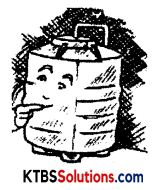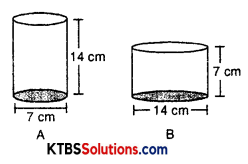# KSEEB Solutions for Class 8 Maths Chapter 11 Mensuration Ex 11.4

## KSEEB Solutions for Class 8 Maths Chapter 11 Mensuration Ex 11.4

Question 1.
Given a cylindrical tank, in which situation will you find the surface area and in which situation volume.
(a) To find how much it can hold.
(b) Number of cement bags required to plaster it.
(c) To find the number of smaller tanks that can be filled with water from it.Solution:
(a) In this situation volume is to find out.
(b) Surface area.
(c) Volume.Question 2.
The diameter of cylinder A is 7 cm, and the height is 14 cm. The diameter of cylinder B is 14 cm and height is 7 cm. Without doing any calculations can you suggest whose volume is greater? Verify it by finding the volume of both cylinders. Check whether the cylinder with greater volume also has greater surface area?Solution:
Cylinder B has a greater volume
Radius of cylinder (A) = $$\frac{7}{2}$$ cm
Height of cylinder = 14 cm
Volume of cylinder (A) = πr2h
= $$\frac{22}{7} \times\left(\frac{7}{2}\right)^{2} \times 14$$
= $$\frac{22}{7} \times \frac{7}{2} \times \frac{7}{2} \times 14$$
= 11 × 49
= 539 cm3
Radius of cylinder B = 7 cm
Height of cylinder = 7 cm
Volume of cylinder B = πr2h
= $$\frac{22}{7}$$ × 7 × 7 × 7
= 22 × 49
= 1078 cm3
Surface area of cylinder (A) = 2πrh + 2πr2
= 2πr(r + h)
= $$2 \times \frac{22}{7} \times \frac{7}{2}\left[\frac{7}{2}+14\right]$$
= $$22\left[\frac{7+28}{2}\right]$$
= 11 × 35
= 385 cm2
Surface area of cylinder B = 2πrh + 2πr2
= 2πr(r + h)
= 2 × $$\frac{22}{7}$$ × 7[7 + 7]
= 44 × 14
= 616 cm2Question 3.
Find the height of a cuboid whose base area is 180 cm2 and volume is 900 cm3?
Solution:
Volume of cuboid = 900 cm3
⇒ (Area of base) × height = 900
⇒ 180 × height = 900
⇒ height = $$\frac{900}{180}$$ = 5 cm
Hence, height of cuboid = 5 cm

Question 4.
A cuboid is of dimensions 60 cm × 54 cm × 30 cm. How many small cubes with a side of 6 cm can be placed in the given cuboid?
Solution:
Volume of cuboid = 60 × 54 × 30
= 1800 × 54
= 97200 cm3
Edge of cube = 6 cm
Volume of cube = (Side)3
= (6)3
= 6 × 6 × 6
= 216 cm3
Number of cubes = $$\frac{\text { Volume of cuboid }}{\text { Volume of cube }}$$
= $$\frac{97200}{216}$$
= 450Question 5.
Find the height of the cylinder whose volume is 1.54 m3 and diameter of the base is 140 cm?
Solution:
Volume of cylinder = 1.54 m3
Diameter of base = 140 cm
radius of base = $$\frac{140}{2}$$
= 70 cm
= $$\frac{70}{100}$$ m
= 0.70 m
πr2h = 1.54
$$\frac{22}{7}$$ × 0.7 × 0.7 × h = 1.54
h = $$\frac{1.54 \times 7}{22 \times 0.7 \times 0.7}$$
= $$\frac{154 \times 7}{22 \times 7 \times 7}$$
= 1
∴ Height of cylinder = 1 mts.

Question 6.
A milk tank is in the form of a cylinder whose radius is 1.5 m and length is 7 m. Find the quantity of milk in litres that can be stored in the tank?Solution:
Radius of cylinder = 1.5 m
Height of cylinder = 7 m
Volume of tank = πr2h
= $$\frac{22}{7}$$ × 1.5 × 1.5 × 7
= 22 × 2.25
= 49.50 m3
1 m3 = 1000 lts
49.5 m3 = 49.5 × 1000 = 49500 ltsQuestion 7.
If each edge of a cube is doubled,
(i) how many times will its surface area increase?
(ii) how many times will its volume increase?
Solution:
Let edge of cube = x unit
(i) Surface area of cube = 6(Side)2 = 6x2
Volume of cube = (Side)3 = x3
If edge of cube is doubled = 2x unit

(ii) Surface area of cube = 6(Side)2
= 6(2x)2
= 6 × 4x2
= 24x2
Volume of cube = (Side)3
= (2x)3
= 8x3
Original surface area = 6x2
Increased surface area = 24x2
Hence, Surface area increased = $$\frac{24 x^{2}}{6 x^{2}}$$ = 4 times increased
Volume increased = $$\frac{8 x^{3}}{x^{3}}$$ = 8 times increasedQuestion 8.
Water is pouring into a cubiodal reservoir at the rate of 60 liters per minute. If the volume of reservoir is 108 m3, find the number of hours it will take to fill the reservoir.Solution:
Volume of reservoir = 108 m3
= 108 × 1000
= 108000 lts
Since, water is pouring into reservoir at the rate of 60 lts per min.
Time taken to fill the reservoir = $$\frac{108000}{60}$$
= 1800 mins
= $$\frac{1800}{60}$$ hrs
= 30 hrs